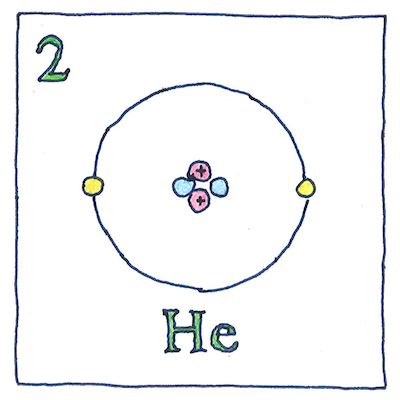# 1911

## The book of science

Tom Sharp

 Antonius van den Broek chemistry

## Atomic number

• Ernest Rutherford realized
• the nucleus of an atom
• has a positive charge
• to be measured in units
• of the charge of an electron;
• however, he guessed
• it would be equal
• to half the atomic weight,
• which Antonius van den Broek
• quickly corrected, claiming
• the charge should be
• exactly equal to its position
• in the periodic table.
• *
• An element’s position
• in the periodic table
• is based on the number
• of protons in its nucleus.
• The number of neutrons
• is not always equal
• to the number of protons;
• many elements have
• multiple isotopes, each
• with a different number
• of neutrons but this
• was not yet understood.

## Atomic parts

• Nucleus
• containing protons and neutrons
• Electrons
• in atomic orbitals
• Electromagnetic force
• between protons and electrons
• Nuclear force
• between protons and neutrons
• Magnetic force
• is related to angular momentums
• of its nucleus and electrons
• and the spin of its electrons.

## Z

• Z for zahl
• in German, “number”
• is shorthand for “atomic number,”
• also for impedance,
• the third axis,
• the end of a series,
• Zoro,
• and, repeated, sleep.

Dmitri Mendeleev tried to order the first periodic table according to ascending atomic weights; however, he had to place tellurium ahead of iodine, even though tellurium has the greater atomic weight, to better align the chemical properties of the two with the chemical properties of the elements above them. The modern periodic table is arranged by atomic number.

Francis William Aston discovered isotopes in 1922, and James Chadwick discovered the neutron in 1932.

See also in The book of science:

Readings in wikipedia: